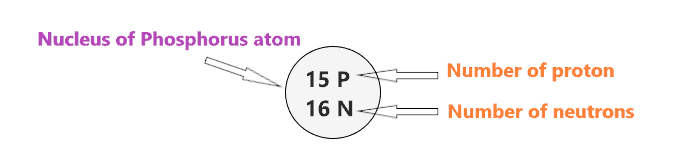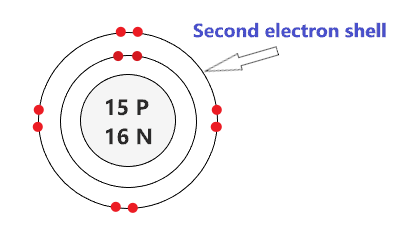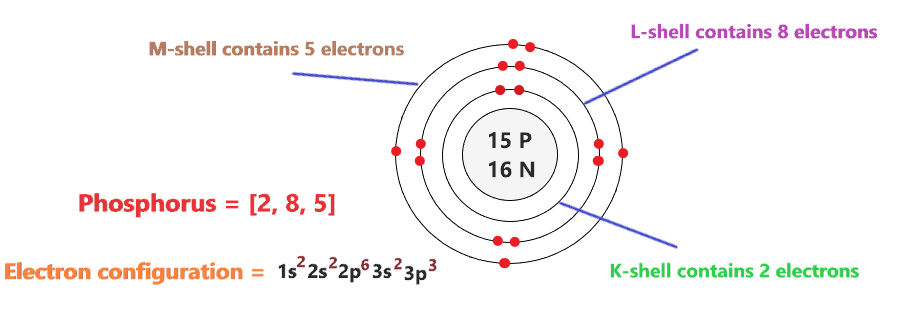# Bohr model of Phosphorus atom - How to draw Phosphorus(P) Bohr-Rutherford diagram?

Home  > Chemistry Article > Phosphorus Bohr model

The Bohr Model of Phosphorus(P) has a nucleus that contains 16 neutrons and 15 protons. This nucleus is surrounded by three-electron shells named K-shell, L-shell, and M-shell. The outermost shell in the Bohr diagram of Phosphorus contains 5 electrons that also called valence electrons.

 Name Phosphorus Bohr Model Number of neutrons 16 Number of protons 15 Number of electrons 15 Total electron shells 3 Electron in the First shell(K) 2 Electrons in the Second shell(L) 8 Electrons in the Third shell(M) 5 Total valence electrons in Phosphorus 5
Page Contents

## How to draw Bohr Model of Phosphorus(P)?

Bohr model describes the visual representation of orbiting electrons around the small nucleus. It used different electron shells such as K, L, M, N…so on. These shells hold a specific number of electrons, the electron shell which is closest to the nucleus has less energy and the electron shell which is farthest from the nucleus has more energy.

Bohr diagram is very interesting and easy to draw. Here, we will draw the Bohr diagram of the Phosphorus atom with some simple steps.

## Steps to draw the Bohr Model of Phosphorus atom

1. Find the number of protons, electrons, and neutrons in the Phosphorus atom

Protons are the positively charged particles and neutrons are the uncharged particles, both these are constituents of the atom nuclei. Electrons are the negatively charged particles that orbit the nucleus of an atom

To find the number of protons an atom contains, just look at their atomic number.

If the atomic number of atom is 23, then proton will also be 23, if atomic number is 29, then proton will also be 29.

So, the atomic number for Phosphorus is 15, hence, the number of protons in the Phosphorus atom is also 15.Now, to determine the number of neutrons in an atom, use this formula.

⇒ Number of neutrons in atom = Atomic mass of the atom(rounded to the nearest whole number) – Number of proton in an atom

For example, An atom have 41.98 atomic mass and 20 protons.

Then, to find the number of neutron, round the atomic mass to the near whole number, so, atomic mass 41.98 round to 42.

= (42 – 20 protons) = 22 number of neutrons in the atom

Now, To get the number of neutrons in a Phosphorus atom, look at its atomic mass which is 30.97 rounded to 31, and the number of protons in Phosphorus is 15.

∴ Hence, the number of neutrons in Phosphorus atom = (31 – 15) = 16It should be noted that “The number of electrons in a neutral atom is equal to the number of protons”.

So, the Phosphorus atom is neutral, hence, its number of electrons will be equal to the number of protons which is 15 as we already discussed.

⇒ The number of electrons in Phosphorus atom = 15

⇒ The number of protons in Phosphorus atom = 15

⇒ The number of neutrons in Phosphorus atom = 16

2. Draw the nucleus of an atom

A nucleus is a dense and small region that contains the number of protons and neutrons of an atom.

In this step, we have to draw a small circle that consists of a number of protons and the number of neutrons of a Phosphorus atom.3. Draw the First electron shell

“An electron shell may be thought of as an orbit followed by electrons around an atom’s nucleus.”

The first electron shell is also called the K-shell, this is the closest shell to the nucleus of an atom and can hold a maximum of two electrons.

As we know, the Phosphorus atom has a total of 15 electrons. So, put two electrons from it, in the first shell, next to each other.We have successfully drawn the first shell of the Phosphorus atom that can hold 2 electrons. As a Phosphorus atom has a total of 15 electrons, and from 15 electrons we have used two electrons in the first shell.

∴ (15 – 2) = 13 electrons

Therefore, we are left with 13 electrons, let’s put them in the next shells of the Phosphorus atom.

4. Draw the Second electron shell

The second shell also called the L-shell that can hold a maximum of 8 electrons. This shell is drawn after the first electron shell.

In the second electron shell, the electrons are added one at a time, starting from the top position then going in a clockwise direction.

In second shell, electrons are added one at a time in clockwise direction as a clock position – 12 o’clock, 3 o’clock, 6 o’clock, 9 o’clock positions.Once you place the electrons one at a time to each of the four sides(Top – Right – Bottom – Left], start pairing or doubling them.

So, we have 13 remaining electrons of a Phosphorus atom, and the 2nd shell can only hold a maximum of 8 electrons.

Therefore, put the 8 electrons of the Phosphorus atom in the 2nd electron shell, start from the top position, put electrons one at a time, and go in a clockwise direction(Top – Right – Bottom – Left). And, finally, pair them up.As we have a total of 15 electrons for the Phosphorus atom and we placed 2 electrons in the first shell and 8 electrons in the second shell, hence, we are left with 5 electrons more.

Let’s place it in the next shell.

5. Draw the Third electron shell

The third shell is also called the M-shell and it has the capacity to hold up to 18 electrons but, for the elements in the first few periods, the third shell only holds up to 8 electrons.

“The third shell can be considered to hold 8 or 18 electrons but in total the third shell can hold 18 electrons.”

For the first few elements, say, till atomic number 20 on the periodic table, the third shell holds up to 8 electrons.

⇒ For atomic number 20 or less than 20, the third shell can hold up to maximum of 8 electrons.

⇒ For the atomic number more than 20, say, atomic numbers 21, 22, 23, 24, 25, 26, 27,…….etc., the third shell is filled with more than 8 electrons and up to maximum of 18 electrons.

For the Phosphorus atom, the third shell is filled with the same procedure as the second shell.

So, we have left with only 5 electrons of a Phosphorus atom, so, start from the top position, put electrons one at a time, and go in a clockwise direction(Top – Right – Bottom – Left). And, finally, start pairing.### Bohr model of Phosphorus atom

That’s all, this is our Bohr model of the Phosphorus atom that contains 15 protons and 16 neutrons in the nucleus region, and 15 electrons are orbited around the nucleus, two electrons in the first shell, eight electrons in the second shell, and five electrons in the third shell.

## Find Valence electron of Phosphorus through its Bohr diagram

From the Bohr diagram of an atom, we can easily find the number of valence electrons in an atom by looking at its outermost shell.

So, we have to find a valence electron in the Phosphorus atom, for this, look at its Bohr diagram.

Bohr’s diagram of Phosphorus has three electron shells (K, L, and M), the inner shell is K-shell and the outermost shell is M-shell.

Hence, the electrons found in the M-shell of the Phosphorus atom are its valence electrons because it is the outermost shell that also called the valence shell.

The M-shell or outer shell of the Phosphorus Bohr model contains only 5 electrons, therefore, the number of the valence electrons in the Phosphorus atom is also 5.## Electron dot diagram of a Phosphorus atom

Electron dot diagram also called lewis structure which represents the valence electrons of atoms.

As, from the Bohr diagram of Phosphorus, we got to know, it has 5 valence electrons.

So, just represent the 5 valence electrons around the Phosphorus atom as a dot.## The electron configuration of Phosphorus

Phosphorus has an atomic number of 15 and it contains a total number of 15 electrons. From the Bohr model of Phosphorus, we know, 2 electrons are in the K-shell, 8 electrons are in the L-shell, and 5 electrons are in the M-shell.

So, based on the shell, the electronic configuration of the Phosphorus atom is [2, 8, 5].

Or the electronic configuration of Phosphorus is 1s22s22p63s23p3 since it contains a total of 15 electrons.## FAQ

### How many electron shells a Phosphorus Bohr model contains?

Electron shell also called energy level, you can find the number of electron shells for an element by knowing its period number in the periodic table.

The elements or atoms in the first period of the periodic table have one energy level or one electron shell, same as, the elements in the second period have two energy levels or two-electron shells and so on…

So, the Phosphorus atom belongs to the 3rd Period in the periodic table, hence, the number of electron shells for the Bohr model of Phosphorus will be 3 (K-shell, L-shell, and M-shell).

### What is the outer shell of the Bohr diagram of the Phosphorus atom?

The outermost shell also called the valence shell, which contains valence electrons of the atom.

According to the Bohr diagram of Phosphorus, the outer shell is M-shell which contains five valence electrons.

## Properties of Phosphorus

1. It exists in two major forms, red phosphorus, and white phosphorus.
2. It is highly reactive and flammable in nature.
3. It has a body-centered cubic crystal structure.
4. It is never found as a free element on Earth.
5. Phosphorus is a weak acid in nature.

## Summary

• The Bohr model of Phosphorus(P) is drawn with three electron shells, the first shell contains 2 electrons, the second shell contains 8 electrons and the third shell contains 5 electrons.
• Phosphorus is neutral and its atomic number is 15, hence, the number of protons and electrons available for its Bohr diagram is also 15.
• The number of neutrons for the Bohr diagram of Phosphorus can be found by subtracting the number of protons from the atomic mass(rounded to the nearest whole).
• The electron configuration of Phosphorus in terms of the shell is [2, 8, 5], or in normal form, it is [Ne] 3s23p3.
##### Subscribe to Blog via Email

Join 2 other subscribers

Share it...